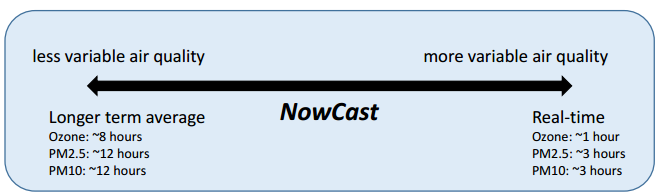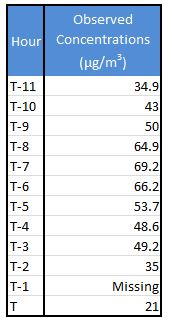# The NowCast for PM2.5 and PM10

## Nowcast Overview

From the EPA’s AQI Technical Assistance Document page 7:

EPA uses the NowCast to approximate the complete daily AQI during any given hour. Even on days when the AQI forecast predicts unhealthy conditions, pollution levels may be lower and better for outdoor activities during some parts of the day. Providing current conditions gives people the power to take action to reduce outdoor activities and exposure when necessary and protect their health.

The NowCast calculation uses longer averages during periods of stable air quality and shorter averages when air quality is changing rapidly, such as during a fire. The NowCast allows current conditions maps to align more closely with what people are actually seeing or experiencing## Completeness Criteria for the NowCast and Clarification on Truncation vs. Rounding

• There is only 1 requirement for a valid NowCast calculation: 2 of the past 3 hourly data points must be valid.

• The Nowcast calculation uses the raw hourly concentrations (not truncated or rounded). The Nowcast values are then truncated to 1 ug/m3 for PM10 or 0.1 ug/m3 for PM2.5. This truncated concentration is then converted to AQI using the AQI equation. The resulting AQI is rounded to the nearest whole number.

## PM2.5 and PM10 NowCast Calculation

The process for calculating the NowCast concentration and AQI for PM2.5 or PM10 is as follows:

1. Compute the concentrations range (max-min) over the last 12 hours.

2. Divide the range by the maximum concentration in the 12 hour period to obtain the scaled rate of change.

3. Compute the weight factor by subtracting the scaled rate from 1. The weight factor must be between .5 and 1. The minimum limit approximates a 3-hour average. If the weight factor is less than .5, then set it equal to .5.

4. Multiply each hourly concentration by the weight factor raised to the power of how many hours ago the concentration was measured (for the current hour, the factor is raised to the zero power).

5. Compute the NowCast by summing these products and dividing by the sum of the weight factors raised to the power of how many hours ago the concentration was measured.

Example Calculation (PM2.5)1. Range: 69.2-21=48.2
2. Scaled rate of change: 48.2/69.2= .69653…
3. Weight Factor: 1-.69653…= .30347… The weight factor for PM2.5 and PM10 must be between 0.5 and 1, so set weight factor to 0.5
4. 21*(.5)0+Missing*(.5)1+35*(.5)2+49.2*(.5)3+48.6*(.5)4+53.7*(.5)5+66.2*(.5)6+69.2*(.5)7+64.9*(.5)8+50*(.5)9+43*(.5)10+34.9*(.5)11= 42.60008…
5. 42.60008…/ [(.5)0+(.5)1+(.5)2+(.5)3+(.5)4+(.5)5+(.5)6+(.5)7+(.5)8+(.5)9+(.5)10+(.5)11]=